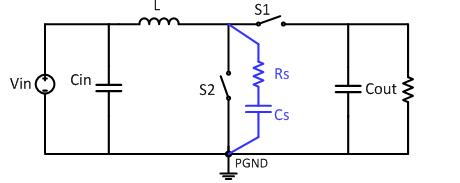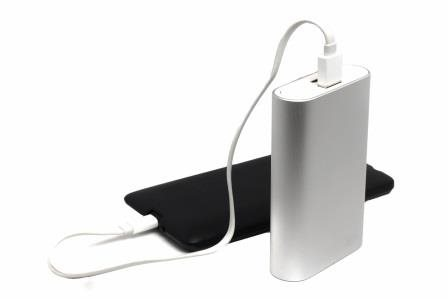A key design challenge when designing a power bank is passing the electromagnetic interference (EMI) test. Electronics engineers often fear EMI test failures, and it would be a nightmare if the circuit failed the EMI test again and again. You would have to work day and night in the lab to fix the problem to avoid product launch delays. For consumer products like power banks, the design period is short, while the EMI certification limit is strict. So you want to add enough EMI filters to pass the EMI test smoothly, but you also don’t want to increase space or add much cost to the circuit. It seems hard to achieve both.

The Low Radiated EMI Boost Converter Reference Design provides such a solution. It can support a 2.7V-4.4V input voltage, 5V/3A, 9V/2A and 12V/1.5A output power, making it fit for the power bank applications. By optimizing the placement and layout, this reference design can get higher than 6dB margin in EN 55022 and International Special Committee on Radio Interference (CISPR) 22 Class-B radiation tests. Let’s take a look at the design process.

Identify the critical current path

EMI starts off from the high instantaneous rate of current change (di/dt) loops. So you should differentiate the high di/dt critical path at the beginning of your design. It is important to understand the current conduction paths and signal flows in the switching power supply.

Figure 1 shows the topology and critical current path of the boost converter. When S2 closes and S1 opens, AC current flows through the blue loop. When S1 closes and S2 opens, AC current flows through the green loop. So the current flow through input capacitor Cin and inductor L is a continuous current, while the current flow through S2, S1 and the output capacitor Cout is a pulsating current (red loop). The red loop is thus the critical current path. This path has the highest EMI energy. You should minimize the area enclosed by it during layout.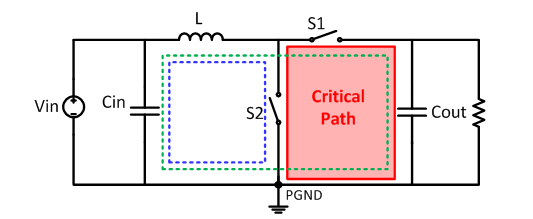Figure 1: Critical current path of boost converter

Minimize High di/dt Path Loop Area

Figure 2 shows the pin configuration of the TPS61088. Figure 3 shows the TPS61088 critical current path layout example. The NC pin means that there is no connection inside the device, so it can be connected to PGND. From an electrical point of view, connecting the two NC pins to the PGND ground plane is good for thermal dissipation and can reduce the impedance of the return path. From an EMI point of view, connecting two NC pins to the PGND ground plane makes the VOUT and PGND plane of the TPS61088 much closer to each other. And that makes the placement of the output capacitors much easier.

From Figure 3, you can see that placing one 0603 1µF (or 0402 1µF) high-frequency ceramic capacitor COUT_HF as close to the VOUT pin as possible results in a minimum area of the high di/dt loop.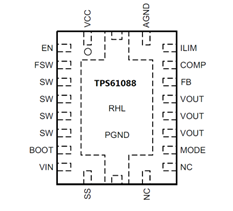Figure 2: TPS61088 pin configuration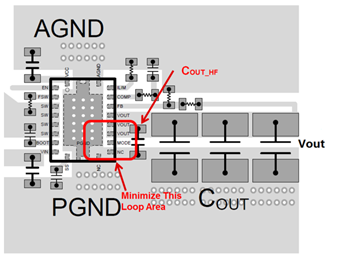Figure 3: TPS61088 critical path layout example

Equation 1 calculates the maximum electric field strength from such a high di/di loop over a ground plane at a 10m distance: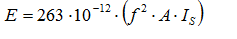Where A is the loop area,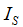is the current flowing in the loop,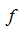is the interested frequency of . So smaller critical path area means smaller radiation energy.

Figure 4 shows the radiated EMI result with and without COUT_HF. Under the same test condition, the radiated EMI is improved by 4dBuV/m with COUT_HF.

Figure 4: Radiated EMI result with and without COUT_HF

Putting a ground plane under the critical path

High trace inductance leads to poor radiation EMI because the magnetic field strength is in direct proportion to the inductance. Placing a solid ground plane on the next layer of the critical trace can solve this problem.

Table 1 gives the inductance of a given trace on different printed circuit board (PCB) boards. You can see that for a four-layer PCB with a 0.4mm insulation thickness between the signal layer and the ground plane, the trace inductance is much smaller than that of a 1.2mm-thick two-layer PCB. So putting a solid ground plane with a minimum distance to the critical path is one of the most effective ways to reduce EMI.

Table 1. Trace inductance (trace length = 5cm)

 PCB h (mm) Wg(mm) L(nH) Single-Layer PCB -- -- 52 2-Layer PCB 1.2 10 3.6 4-Layer PCB 0.4 10 1.2

Figure 5 shows the radiated EMI result of a two-layer PCB and a four-layer PCB. Under the same layouts and same test conditions, the radiated EMI improves by more than 10dBµV/m with a four-layer PCB.

Figure 5: Radiated EMI result of a two-layer PCB and a four-layer PCB